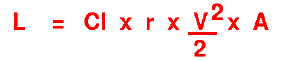+ Text Only Site
+ Non-Flash Version
+ Contact Glenn### Lift Equation in its Y = mX + b Form ActivityIf so instructed by your teacher, print out a worksheet page for these problems.

The Lift EquationLift = lift coefficient x air density x velocity squared / two x wing area

The lift coefficient, Cl, contains all the complex dependencies and is usually determined experimentally.

For thin airfoils with camber and at angle of attack:

Cl = 2 p a + Clo

( p = 3.1416) and ( a is in radians)

NOTICE: This equation holds true only at small angles of a. ( [a] < 10 degrees)

1a. Identify each letter in the lift equation and list the units for each.

Cl = _________________

L = _________________

r = _________________

V = ________________

A = ________________

1b. Identify each letter in the thin airfoil equation and list the units for each.

Cl = _________________

a = _________________

Clo = _________________

2. Write out two equations for Cl (one from the lift equation and the other from the thin airfoil equation).

3. Combine the two equations into one equation that shows L as a function of a. Notice that this is in the form of y = mx + b, where y is now L , x is a , and m and b are combinations of the other variables.

4. Now use FoilSim to determine Clo. On FoilSim set units to Metric Units. Set the camber to 10% the angle to 0.0, and the area to 2 sq m. Record the values shown for lift (L) , density (r) , and the other input values. Then use the equation from #3 to solve for Clo.

5. The value of Clo (constant) you solved for in #4 can now be used to solve for the lift as a function of the angle of attack by graphing methods. Make a graph using your equation from #3 and using angles ( a ) of -20, -15, -10, -5, 0, 5, 10, 15, 20 (changed to radians). Notice that when a = zero, the lift is NOT zero. It is the y intercept (b).

6. Verify you answers using Foilsim. Use the same angles and record the lift values. Graph these results in a different color on your graph. Are the lines the same? If they are different, what do you think causes them to be different?

7. Use FoilSim and replace the area of the wing with a different value. Report what happens to the lift.

Related Pages:
Standards
Worksheet
Lesson Index
Aerodynamics Index+ Inspector General Hotline + Equal Employment Opportunity Data Posted Pursuant to the No Fear Act + Budgets, Strategic Plans and Accountability Reports + Freedom of Information Act + The President's Management Agenda + NASA Privacy Statement, Disclaimer, and Accessibility CertificationEditor: Tom Benson NASA Official: Tom Benson Last Updated: Thu, May 13 02:38:38 PM EDT 2021 + Contact Glenn